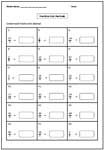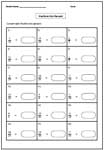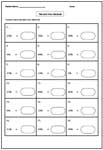1. Worksheets>
2. Math>
3. Pre-Algebra>
4. Convert Between Numbers

# Converting Fractions, Decimals, Percent Worksheets

The printable worksheets in this page include practice skills in converting between fraction, decimal and percent. This page is broadly classified into four major sections, with three sections about converting into different forms and one section is based on multiple choice questions. Thumb through some of these worksheets for free!

## Fraction to Decimal and Percent

Fraction to Decimal: Basic

The denominators of fractions are multiples of 10s.Fraction to Decimal: Standard

Divide numerator with denominator to convert each fraction into decimal. Answers are terminating decimals.Fraction to Percent

Convert each fraction into percent. To convert into percent, multiply the numerator of the fraction with 100, and divide the product with denominator.Mixed Review

Each printable worksheet include two sections, first section about converting fraction into decimal and the next about converting into percent.## Decimal to Fraction and Percent

Decimal to Fraction

Write each decimal to fraction in simplest form.Easy:

Moderate:

Decimal to Percent

Basic practice skills in converting decimal to percent are enclosed in these pdf worksheets.Mixed Review

Mixed review pdfs include converting decimals into both fractions and percent.## Percent to Fraction and Decimal

Percent to Fraction

Basic practice skills in converting percent into fraction.Percent to Decimal

Basic practice skills in converting percent into decimal.## Multiple Choice Questions

You have four options to choose. Choose the correct choice that is equivalent to either fraction or decimal or percent.Fraction to Decimal or Percent:

Decimal to Fraction or Percent:

Percent to Fraction or Decimal:

Mixed Review: All 3 forms

Related Worksheets